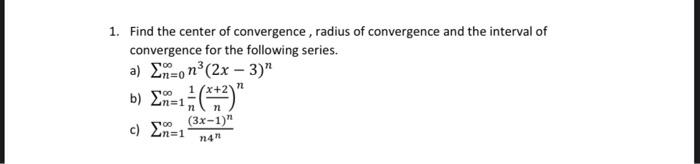### Create an Account

Already have account?

### Forgot Your Password ?

Home / Questions / 1. Find the center of convergence , radius of convergence and the interval of convergence ...

# 1. Find the center of convergence , radius of convergence and the interval of convergence for the following series. a) Enzo n3 (2x - 3)" n 1/x+2 b) En=1 c) En=1 oo n (3x-1)" n4

1. Find the center of convergence , radius of convergence and the interval of convergence for the following series. a) Enzo n3 (2x - 3)" n 1/x+2 b) En=1 c) En=1 oo n (3x-1)" n4Apr 15 2021 View more View Less

#### Answer (Solved)Subscribe To Get Solution